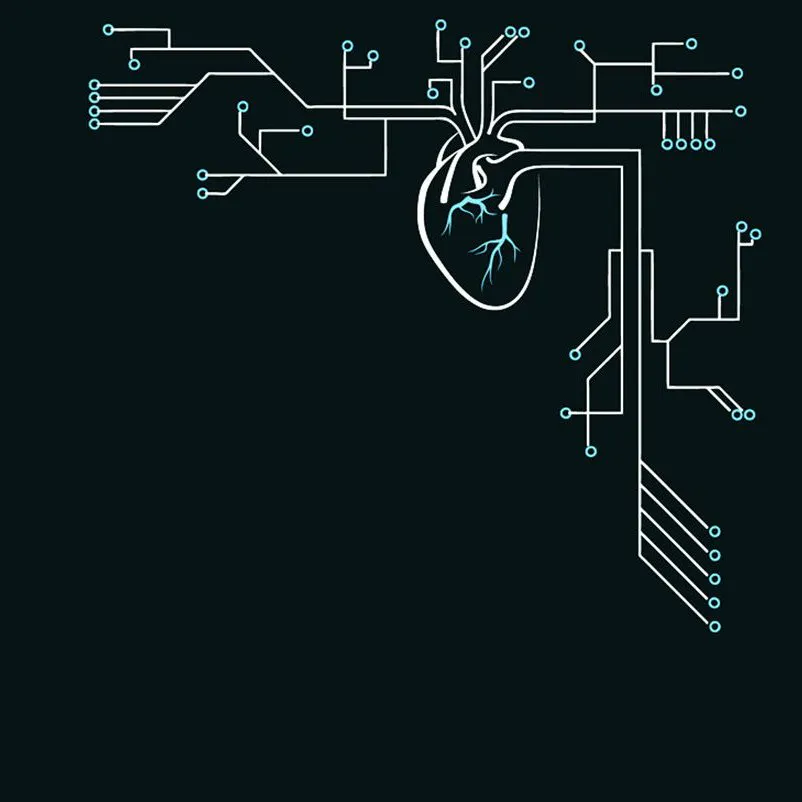Published

Fear not ol' forgetful geezers for I have a solution for the ever-existing problem in these pandemic time of forgetting face mask!!

BeginnerShowcase (no instructions)58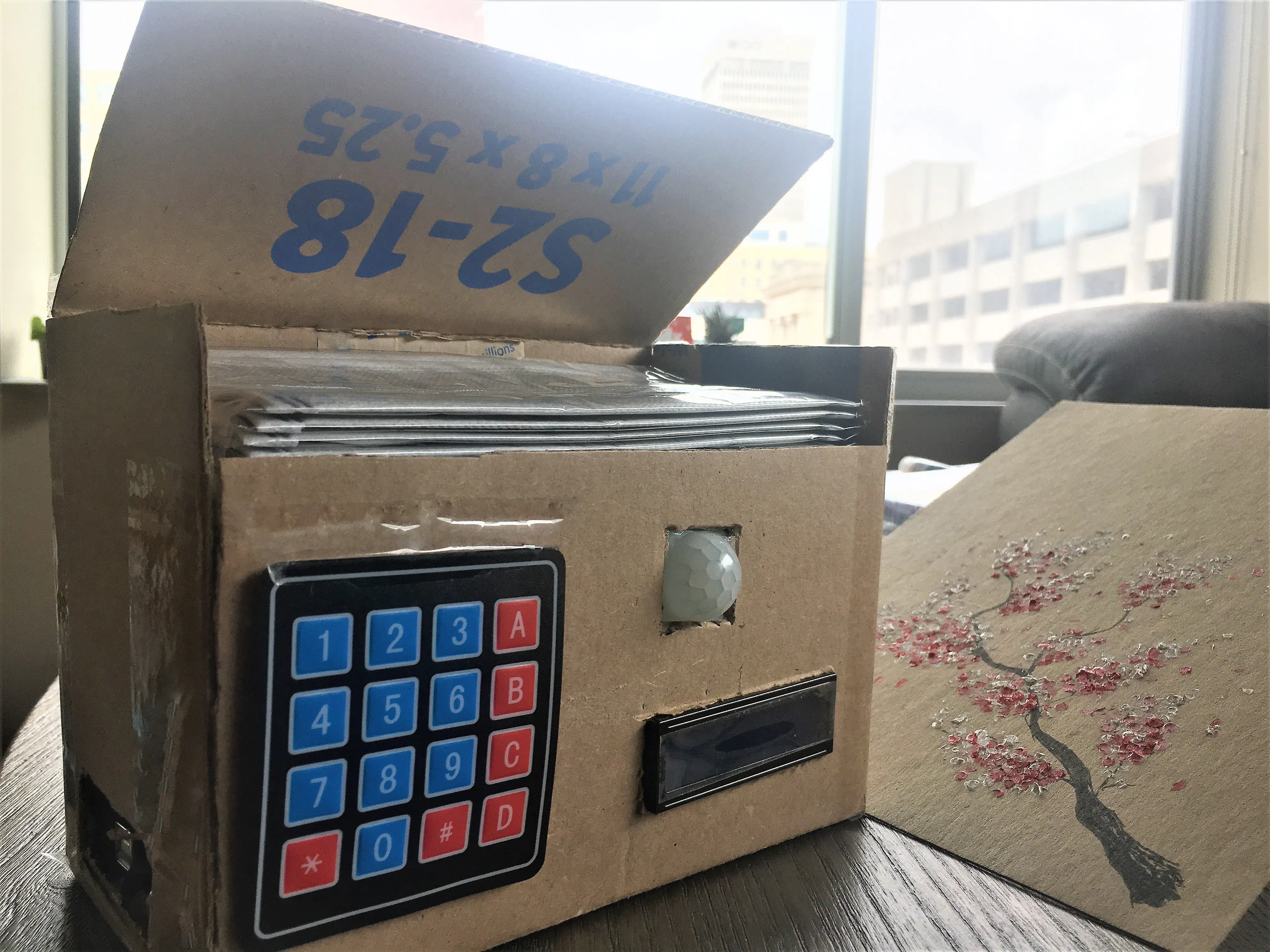## Things used in this project

### Hardware componentsArduino UNO & Genuino UNO
×1Jumper wires (generic)
×1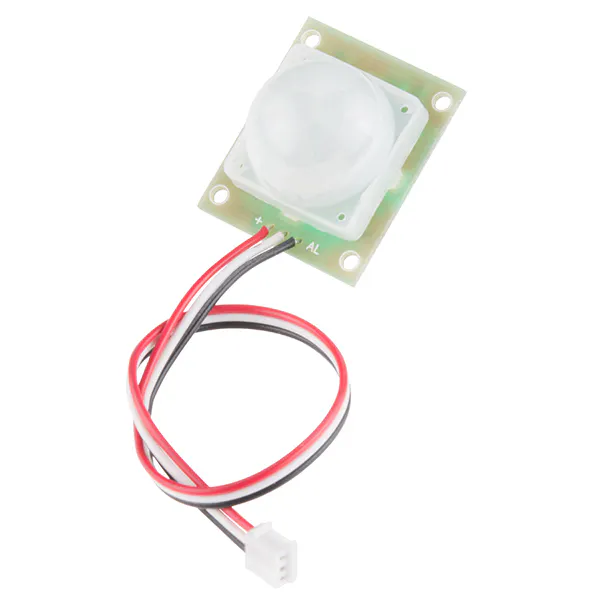PIR Motion Sensor (generic)
×1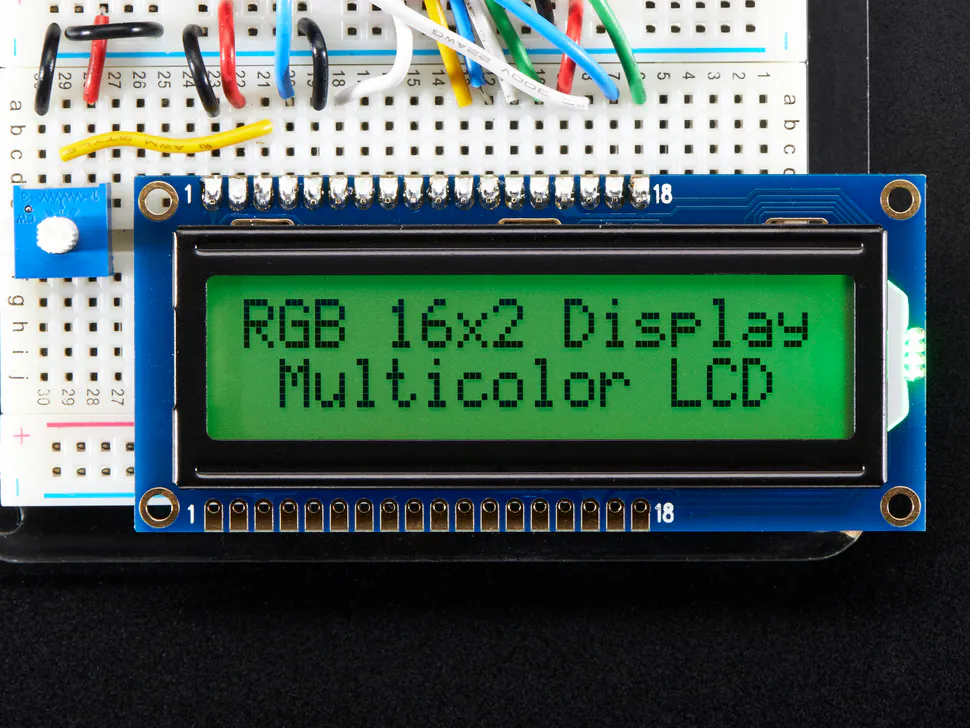Adafruit RGB Backlight LCD - 16x2
×1×1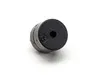Buzzer
×1

### Software apps and online servicesArduino IDE

## Code

Arduino
```#include <LiquidCrystal.h>

LiquidCrystal lcd(12,11,A4,A5,13,10);
const byte ROWS = 4;
const byte COLS = 4;
const int pirPin = A3;
char keys[ROWS][COLS] = {
{'7','8','9','C'},
{'1','2','3','A'},
{'4','5','6','B'},
{'*','0','#','D'}
};
byte rowPins[ROWS] = { 2, 3, 4, 5 };
byte colPins[COLS] = { 6, 7, 8, 9 };

int fm = 0;

void setup() {
Serial.begin(9600);
lcd.begin(16, 2);
pinMode(pirPin, INPUT);
}

void loop() {
//case 1: when there is no face mask
int fm_temp = 0;
while(fm==0){
lcd.setCursor(0,0);
lcd.setCursor(0,1);
lcd.print("Max 100: ");
char key = kpd.getKey();
if(key){
if(key == '0') fm_temp = fm_temp * 10 + 0;
if(key == '1') fm_temp = fm_temp * 10 + 1;
if(key == '2') fm_temp = fm_temp * 10 + 2;
if(key == '3') fm_temp = fm_temp * 10 + 3;
if(key == '4') fm_temp = fm_temp * 10 + 4;
if(key == '5') fm_temp = fm_temp * 10 + 5;
if(key == '6') fm_temp = fm_temp * 10 + 6;
if(key == '7') fm_temp = fm_temp * 10 + 7;
if(key == '8') fm_temp = fm_temp * 10 + 8;
if(key == '9') fm_temp = fm_temp * 10 + 9;
if(key == 'A') fm_temp = fm_temp / 10;

lcd.setCursor(9,1);
lcd.print(fm_temp);
lcd.print(" ");
if(key == 'D'){
//if number exceeds 100, reset fm to -1
if(fm_temp > 100){
fm = -1;
}else{
fm = fm_temp;
}
fm_temp = 0;
}
}
}
//case 2: when there is one or more face mask
while(fm>0){
lcd.setCursor(0,0);
lcd.print("Current amount:");
lcd.setCursor(0,1);
lcd.print(fm);
lcd.print("             ");
//algorithm for each time motion sensor detects something
if(pirStat == HIGH){
Serial.print("I got chu");
tone(A1, 100, 500);
delay(5000);
if(fm > 1){
fm--;
}else if(fm == 1){
fm = -2;
}
}
}
//case 3: when fm exceeds 100 and need to be reset
while(fm==-1){
lcd.setCursor(0,0);
lcd.print("Invalid number!");
lcd.setCursor(0,1);
lcd.print("Any key to redo ");
char key = kpd.getKey();
if(key){
fm = 0;
}
}
//case 4: when fm runs out and needs to be refilled
while(fm ==-2){
lcd.setCursor(0,0);
lcd.setCursor(0,1);
lcd.print("Any key to input");
char key = kpd.getKey();
if(key){
fm = 0;
}
}
}
```

## Credits

### Hung-Che

2 projects • 7 followers
High School Junior